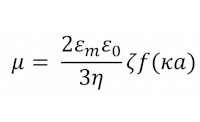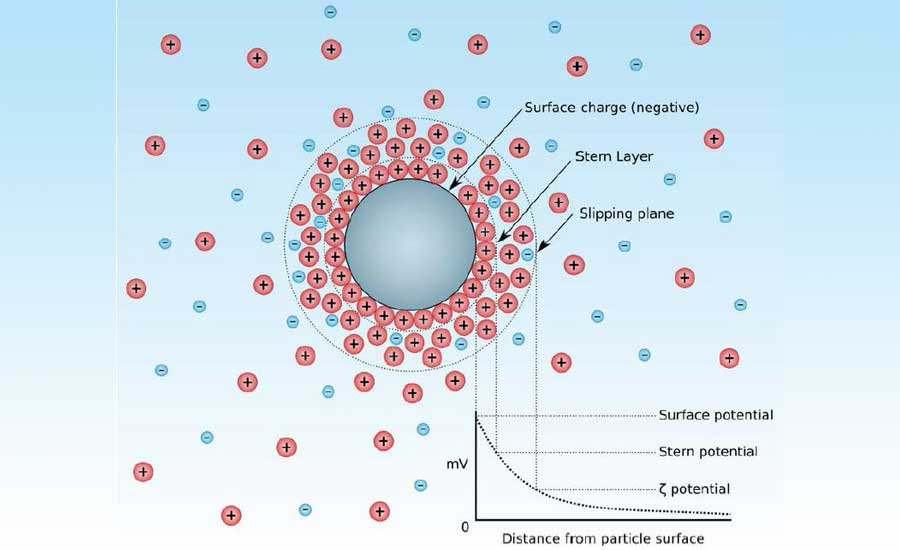Zeta potential (ζ) is a key indicator of the stability of colloidal dispersions. The higher the magnitude of the zeta potential (that is, highly positive OR highly negative), the more stable the colloid. A lower-magnitude zeta potential indicates a less stable colloid; in other words, a tendency towards aggregation or coagulation. Thus, knowledge of zeta potential is important for optimizing processes and for quality control, especially in industries such as pharmaceutical, food and beverages, chemicals, and more.

Frequently, the zeta potential of particles and macromolecules in solution is determined by electrophoretic light scattering (ELS), which is also called laser Doppler electrophoresis. It measures the speed of the particles (= electrophoretic mobility, µ) within a defined electric field. The magnitude of the zeta potential indicates the degree of electrostatic repulsion between particles in dispersion. Each particle in solution exhibits a zeta potential due to its surface charge. Zeta potential, however, cannot be measured directly; therefore it must be calculated from the electrophoretic mobility (µ), which can be measured directly.

Each particle has charges distributed on its surface, which could be negative, positive, or a mixture of both. At the interface between the solid surface and the solvent, a double layer is formed which is called the electrical double layer. This happens because charges on the solid surface attract oppositely charged ions in the surrounding liquid. The zeta potential is then the result of the net electric charge within the interfacial double layer. It is proportional to the potential of the surface (surface potential ψ) and this is described by the theory of the Stern layer (see Figure 1).

An important parameter regarding zeta potential is pH. Lowering the pH by adding acid means adding positive charges to or neutralizing negative charges on the surface, which results in a more positive (or less negative) zeta potential. Raising the pH by adding base results in a more negative (or less positive) zeta potential. A useful piece of information gained from such measurements is the isoelectric point, which is defined as the pH value where the zeta potential is zero and the particles carry no net charge. Below their isoelectric point particles carry a net positive charge; above their isoelectric point they carry a net negative charge. Close to the isoelectric point, where zeta potential is zero, dispersions are often unstable because the electrostatic repulsion is small. Attractive forces between particles may exceed this repulsion and the dispersion may break and flocculate. Colloids with high-magnitude zeta potential (in general, greater than +/- 30 mV) are electrostatically stabilized while colloids with low-magnitude (closer to zero; i.e., between -20 mV and +20 mV) zeta potentials tend to coagulate or flocculate.

### Electrophoresis and Electrophoretic Mobility

Electrophoresis is defined as the movement of charged particles within a defined electric field. The simplest system is a cell filled with a dispersion and equipped with a pair of electrodes to which a voltage is applied. The particles move to the electrode of opposite charge to the particles’ surface charge. The velocity of the particle movement is proportional to the magnitude of their charge. There are various methods for measuring the velocity of the particles in the electric field (= electrophoretic mobility). One method would be video microscopy, although it is limited to large particle sizes. Electrophoretic light scattering, on the other hand, is also applicable to particle sizes down to a few nanometers.

The zeta potential can be calculated from the electrophoretic mobility by using the Henry equation.µ.. electrophoretic mobility (m2/Vs)

ε……….dielectric constant (F/m)

ζ……….zeta potential (V)

f(κa)….Henry’s function

η……….viscosity (Pa.s)

### Electrophoretic Light Scattering

A laser beam passes through the sample, irradiates the particles dispersed in it, and is scattered by the particles. Because of the applied electric field, the particles are moving. The frequency of the scattered light is slightly shifted because of the Doppler effect. Electrophoretic light scattering determines the velocity of the particle movement by measuring the Doppler shift. The shift frequencies are rather small (a few to several hundred Hertz), and such small frequency shifts cannot be measured directly. The optical system ideally suited for such a measurement is an interferometer. In interferometry, the light that is frequency shifted is combined with a portion of the incident light, the so-called reference beam. When the scattered light and the reference beam interfere, the result is a beat. The frequency of the beat is the frequency difference between the two underlying beams, which is the Doppler shift induced by the particles.

In practice, however, there is an additional problem. With a simple interferometer it is impossible to determine the sign of the Doppler shift and thus the sign of the zeta potential. The measured beat frequency is always positive, no matter whether the Doppler shift is positive or negative. To overcome this problem, modern instruments implement a frequency modulator in the reference beam. This modulator causes a controlled Doppler shift at the frequency of the reference light.

This means that the measured Doppler shift is the sum of two contributions, the Doppler shift induced by the modulator plus the Doppler shift induced by the motion of the particles. Imagine particles without charge, which would then not move at all in the electric field. For such samples, the scattered light is unshifted and the measured beat is solely the modulation frequency. For charged particles, the measured beat frequency increases or decreases, depending on the direction of the particle motion. Thus, the use of the modulator makes it possible to determine the sign of the zeta potentialFigure 1. Diagram showing the ionic concentration and potential difference as a function of distance from the charged surface of a particle suspended in a dispersion medium. The zeta potential is the potential at the slipping plane.

### Continuously Monitored Phase-Analysis Light Scattering

The electrophoretic mobility of particles in non-polar solvents can be very small which results in a very small Doppler shift frequency. On the other hand for solvents with a high ionic strength only a very small field can be applied due to Joule-Thompson heating which also results in small Doppler frequency shifts.

Phase analysis light scattering (PALS) is a method for evaluating electrophoretic light scattering measurements and greatly improves their sensitivity. In PALS, the rate of phase change of the measured interference between light scattered by the sample and the modulated reference beam is analyzed. This rate is compared with a mathematical generated sine wave predetermined by the modulator frequency. Any non-linearity of the modulator and any change in the characteristics of the modulator with time will cause a situation where the mathematically generated sine wave does not reflect the real conditions any more. This practically restricts the application to modulators with small movements and accordingly short measuring intervals.

The continuously monitored PALS is a further development of the classical PALS method with an additional modulator monitor implemented. This modulator monitor detects the interference between modulated and un-modulated laser light. Thus, its beat frequency is solely the modulation frequency and is independent of the electrophoretic motion of the particles. In other words, the frequency difference between sample interference and modulator monitor is exactly the Doppler shift caused by the electrophoretic motion of the particles. Any non-linearity and ageing effects of the modulator are automatically compensated and are not influencing the results when the cmPALS method is used. Thus, the application of cmPALS makes it possible to use modulators with large movements, despite their non-linearity. As a consequence, the novel cmPALS technique ensures that the sensitivity and stability of electrophoretic light scattering measurements for zeta potential determination can be further enhanced, and measurements with shorter duration and with lower electric field applied become feasible without worry of sample degradation.